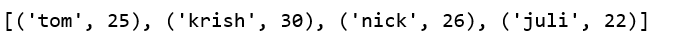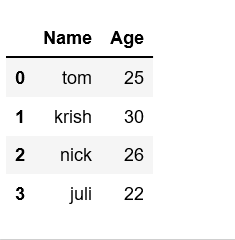# Create pandas dataframe from lists using zip

One of the way to create Pandas DataFrame is by using zip() function.

You can use the lists to create lists of tuples and create a dictionary from it. Then, this dictionary can be used to construct a dataframe.

zip() function creates the objects and that can be used to produce single item at a time. This function can create pandans DataFrames by merging two lists.

Suppose there are two lists of student data, first list holds the name of student and second list holds the age of student. Then we can have,

 `# List1 ` `Name ``=` `[``'tom'``, ``'krish'``, ``'nick'``, ``'juli'``] ` ` `  `# List2 ` `Age ``=` `[``25``, ``30``, ``26``, ``22``] `

Above two lists can be merged by using `list(zip())` function. Now, create the pandas DataFrame by calling `pd.DataFrame()` function.

 `# Python program to demonstrate creating ` `# pandas Datadaframe from lists using zip. ` ` `  `import` `pandas as pd ` ` `  `# List1 ` `Name ``=` `[``'tom'``, ``'krish'``, ``'nick'``, ``'juli'``] ` ` `  `# List2 ` `Age ``=` `[``25``, ``30``, ``26``, ``22``] ` ` `  `# get the list of tuples from two lists. ` `# and merge them by using zip(). ` `list_of_tuples ``=` `list``(``zip``(Name, Age)) ` ` `  `# Assign data to tuples. ` `list_of_tuples  `

Output:`# Converting lists of tuples into ` `# pandas Dataframe. ` `df ``=` `pd.DataFrame(list_of_tuples, columns ``=` `[``'Name'``, ``'Age'``]) ` `  `  `# Print data. ` `df `

Output:My Personal Notes arrow_drop_upCheck out this Author's contributed articles.

If you like GeeksforGeeks and would like to contribute, you can also write an article using contribute.geeksforgeeks.org or mail your article to contribute@geeksforgeeks.org. See your article appearing on the GeeksforGeeks main page and help other Geeks.

Please Improve this article if you find anything incorrect by clicking on the "Improve Article" button below.

Article Tags :

Be the First to upvote.

Please write to us at contribute@geeksforgeeks.org to report any issue with the above content.